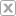# Video Gallery

## Math 7 - Simplifying a Complex Ratio

Go to Video Gallery Added Dec 11, 2020Copy this URL: Embed code:
Go
Simplifying a Complex Ratio aka a Complex Fraction. A Complex Ratio is a Ratio to a Ratio To Simplify a Complex Ratio, convert the complex ratio into a division expression The division expression will be dividing two fractions Dividing two fractions is to convert the division into multiplication and multiply by the Reciprocal aka the Multiplicative Inverse
Show Transcript